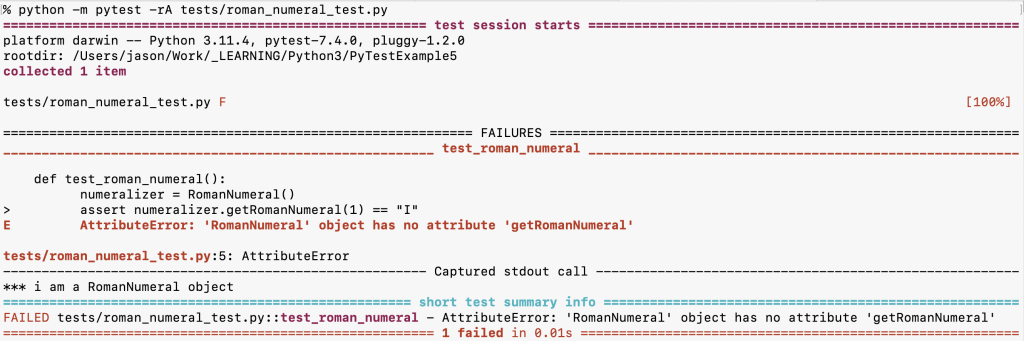# A Pure Refactor with Python

This blog post accompanies my article Automated Testing at 10,000 Feet, which is a broad overview of automated testing and TDD. We’ll make a very simple module to convert Hindu-Arabic numbers (the normal, ten-digit numbers we are used to like 1,2,3,4 ) into Roman numbers (like I, II, III, and IV).

### EXAMPLE 2: A Basic Unit Test in Python

First, make a new directory with `mkdir PyTestExample` and cd into your new directory.

Next, run `pip install pytest` and `mkdir tests`.

If you are going to set up GIt for this repo (recommended), do it now with `git init` and then create a `.gitignore` file and add `__pycache__` to it.

`touch "__pycache__" << .gitignore`

Then, create a new empty file by running `touch tests/__init__.py`. (This file can be empty.)

Then, create a new file at `tests/roman_numeral_test.py`

``````

def test_roman_numeral():
assert True
``````

Run the test with `python -m pytest tests/roman_numeral_test.py`

You’ll see the test pass with:

At the top of the `tests/label_test.py` file, add this line:

`from roman_numeral import RomanNumeral`

Here, we are telling the test to import a module named `roman_numeral` we haven’t yet created.

Now re-run the test and watch it fail because we haven’t yet defined the module `roman_numeral`.

ModuleNotFoundError: No module named ‘roman_numeral’

Remember, the module will be defined by the file name, and the class language construct will define the class. In this example, the module name is `roman_numeral` (lower case and snake case) and the class name will be `Labeler` (upper case and title case)

Next, create a file at the root of the repo called `roman_numeral.py`

In this file, add this code

``````class RomanNumeral:
def __init__(self):
print("*** i am a RomanNumeral object")``````

All that we expect to happen is that when a Labeler object is instantiated, it will print this to the standard output (stdout).

Run the test again, and it will pass again.

But we haven’t created an instance of a RomanNumeral object yet. We’ve just asserted true, which is only for demonstration.

Go back to `tests/roman_numeral_test.py` and remove the line `assert True` and replace it with `numeralizer = RomanNumeral()`

``````from roman_numeral import RomanNumeral
def test_roman_numeral():
numeralizer = RomanNumeral()``````

Now run the test again. You might be expecting to see the output `*** i am a RomanNumeral object ` from the init function, but in fact, we do not yet see it.

That’s because pytest doesn’t show the output unless we pass it special flags, using these flags

So now we’ll run `python -m pytest -rA tests/` to see the output of our print statement.

Notice that our print statement is now shown below the test results. That’s good, because it’s always important to understand how to print and debug when running your tests.

Next, let’s implement a method. First, we’ll write the test:

``````from roman_numeral import RomanNumeral

def test_roman_numeral():
numeralizer = RomanNumeral()
assert numeralizer.getRomanNumeral(1) == "I"``````

Here, we reference a function getRomanNumeral that doesn’t exist yet on our object. So when we run the test we see it fail:p.p1 {margin: 0.0px 0.0px 0.0px 0.0px; font: 16.0px Menlo; color: #b02117; background-color: #f6f6f6} p.p2 {margin: 0.0px 0.0px 0.0px 0.0px; font: 16.0px Menlo; background-color: #f6f6f6; min-height: 19.0px} span.s1 {font-variant-ligatures: no-common-ligatures} span.s2 {font-variant-ligatures: no-common-ligatures; color: #000000}

Here, we see the following error:

E         AttributeError: ‘RomanNumeral’ object has no attribute ‘getRomanNumeral’

Now go back to the RomanNumeral class and add this method:

``````    def getRomanNumeral(self, number):
match number:
case 1:
return "I"``````

Re-run the test and watch it pass now:

Let’s add some more tests to test that our roman numerals work up through 10:

``````def test_roman_numeral():
numeralizer = RomanNumeral()
assert numeralizer.getRomanNumeral(1) == "I"
assert numeralizer.getRomanNumeral(2) == "II"
assert numeralizer.getRomanNumeral(3) == "III"
assert numeralizer.getRomanNumeral(4) == "IV"
assert numeralizer.getRomanNumeral(5) == "V"
assert numeralizer.getRomanNumeral(6) == "VI"
assert numeralizer.getRomanNumeral(7) == "VII"
assert numeralizer.getRomanNumeral(8) == "VIII"
assert numeralizer.getRomanNumeral(9) == "IX"
assert numeralizer.getRomanNumeral(10) == "X"``````

We could easily add this code to make it pass:

``````    def getRomanNumeral(self, number):
match number:
case 1:
return "I"
case 2:
return "II"
case 3:
return "III"
case 4:
return "IV"
case 5:
return "V"
case 6:
return "VI"
case 7:
return "VII"
case 8:
return "VIII"
case 9:
return "IX"
case 10:
return "X"``````

Now that we’ve tested our code, we can do the refactor. From here, we’ll do a “pure refactor” which means we’ll leave our test code in place and only refactor the implementation.

Here’s a simple way to clean up our code a little bit to remove the match / case structure. In this implementation, we’ll create a Python list (called “array” in other languages). The list will hold Roman numerals. Because lists are 0-indexed (meaning the first item in the list will be at index 0), we’ll need to subtract 1 from the number that was input:

``````    def getRomanNumeral(self, number):
romans = ["I", "II", "III", "IV", "V", "VI", "VII", "VIII", "IX", "X"]
return romans[number - 1]``````

Now, we’ve re-implemented the code without changing the tests or adding or removing anything from the feature set. That’s what makes it a “pure refactor.”